# Stellar astronomy, mathematical problems of

(diff) ← Older revision | Latest revision (diff) | Newer revision → (diff)

Problems arising in the investigation of the general laws governing the structure, composition, dynamics, and evolution of stellar systems.

The main type of equation requiring a solution in problems of stellar statistics comprises equations involving the distribution functions of the visible and the true characteristics of objects. As a rule, these are integral equations for the unknown distribution functions of the true characteristics. For example, an important equation for studying the structure of the Galaxy is the Schwarzschild equation(1)

in which the unknown in a given solid angleis the distribution functionof stars over distances, while the distribution function of stars over visible stellar magnitudesand absolute stellar magnitudesare known from observations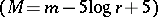. Equation (1) has an exact solution in terms of characteristic functions. The difficulty is that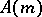is known only up to a finite value of, depending on the limit of telescopic visibility.

Another example is the Abel-type equation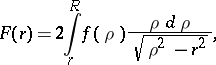relating the observed surface stellar density (here and below, stellar density means the density of the distribution of stars as objects)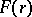of a spherically-symmetric star cluster or galaxy of radiusto the space density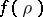.

An example of a two-dimensional integral equation is provided by the equation relating the distribution functions of visible configurations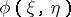and true configurationsof triple stars: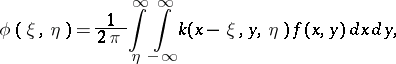wherethis equation rests on the assumption that all orientations of the plane of visible configurations of triple stars are equiprobable;and(and) are the coordinates of the third component of the triple star, providedare the coordinates of the first and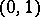those of the second.

A characteristic problem in stellar kinematics is the solution of overdetermined systems of conditional equations, each equation being derived for an individual star or an individual section of the Heavens.

## Contents

### Examples.

1) The system for the determination of the equatorial componentsof the local velocity of the Sun, given observations of the proper motions of stars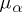and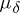, their distancesand the equatorial coordinatesand: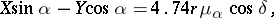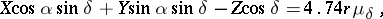and radial stellar velocities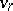: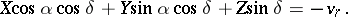2) The system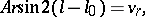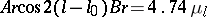for the Oort coefficientsand, characterizing the angular velocity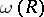of Galactic rotation in the region of the Sun: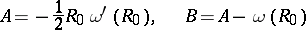and the longitudeof the galactic centre (denotes the galactic longitudes of stars in a vicinity of the galactic equator).

A fundamental equation of stellar dynamics is the Boltzmann equation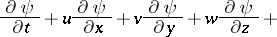(2)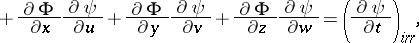whereis the phase density andthe potential of the stellar system. Since a stellar system is self-gravitational, equation (2) must be solved in conjunction with the Poisson equation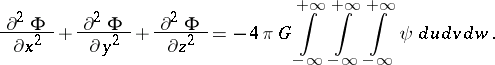If attention is confined to regular forces only (i.e. forces set up by the system as a whole), the right-hand side of (2) vanishes; if irregular forces (arising when the stars of the system approach one another) are also taken into account, the collision integral must also be considered. Examination of the equations (2) and

shows that there are two integrals of motion in spherical systems, and three in rotating systems.

In the hydrodynamic approximation, the hydrodynamic equations derived from (2) are considered.

In the theory of irregular forces of stellar systems, changes in the stellar velocities are often considered as a continuous stochastic process, and one solves the Fokker–Planck equation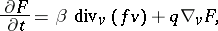where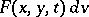is the probability that at timea star has velocityin the interval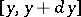, provided its initial velocity was. Hereandare the dynamic friction and diffusion coefficients, respectively, determined by the characteristics of the stellar field.

A more accurate approximation is provided by considering the variation of stellar velocity in the context of a purely discontinuous stochastic process. Once the transition probability density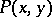and jump probability density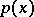have been found, the solution of the Kolmogorov equation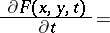(4)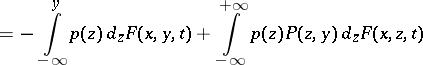determines the function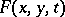. Since stellar systems have a critical velocity, equation (4) is also considered with an absorbent shield.

To investigate the stability of stellar systems, one considers variations of the phase density and of the potential in the Boltzmann equation for equilibrium systems. This yields equations resembling those used in physics to investigate plasma instabilities. Features specific to stellar systems are the self-gravitation and the non-additivity of energy.

The theory of the distribution of the density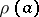in external galaxies requires the solution of the integral equationin which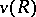is the observed angular velocity of rotation of the galaxy andis the eccentricity of its meridianal section.

How to Cite This Entry:
Stellar astronomy, mathematical problems of. Encyclopedia of Mathematics. URL: http://encyclopediaofmath.org/index.php?title=Stellar_astronomy,_mathematical_problems_of&oldid=12300
This article was adapted from an original article by T.A. Agekyan (originator), which appeared in Encyclopedia of Mathematics - ISBN 1402006098. See original article